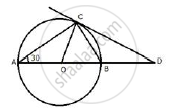Share

Ab is the Diameter and Ac is a Chord of a Circle with Centre O Such that Angle Bac = 30°. the Tangent to the Circle at C Intersects Ab Produced in D. Show that Bc = Bd. - ICSE Class 10 - Mathematics

ConceptTangent Properties - If a Line Touches a Circle and from the Point of Contact, a Chord is Drawn, the Angles Between the Tangent and the Chord Are Respectively Equal to the Angles in the Corresponding Alternate Segments

Question

AB is the diameter and AC is a chord of a circle with centre O such that angle BAC = 30°. The tangent to the circle at C intersects AB produced in D. show that BC = BD.

SolutionJoin OC,
∠BCD = ∠BAC = 30° (angles in alternate segment)
Arc BC subtends ∠DOC at the centre of the circle and ∠BAC at the remaining part of the circle.
∴ ∠ BOC  = 2∠BAC =  2 × 30° = 60°
Now in Δ OCD,
∠BOC or ∠DOC =  60°
∠OCD = 90° (OC ⊥ CD)
∴ ∠DCO+ ∠ODC =  90°
⇒ 60°  + ∠ODC  = 90°
⇒ ∠ODC  = 90° - 60° = 30°
Now in ΔBCD,
∵ ∠ODC or ∠BDC = ∠BCD =  30°
∴ BC = BD

Is there an error in this question or solution?

Video TutorialsVIEW ALL 

Solution Ab is the Diameter and Ac is a Chord of a Circle with Centre O Such that Angle Bac = 30°. the Tangent to the Circle at C Intersects Ab Produced in D. Show that Bc = Bd. Concept: Tangent Properties - If a Line Touches a Circle and from the Point of Contact, a Chord is Drawn, the Angles Between the Tangent and the Chord Are Respectively Equal to the Angles in the Corresponding Alternate Segments.
S